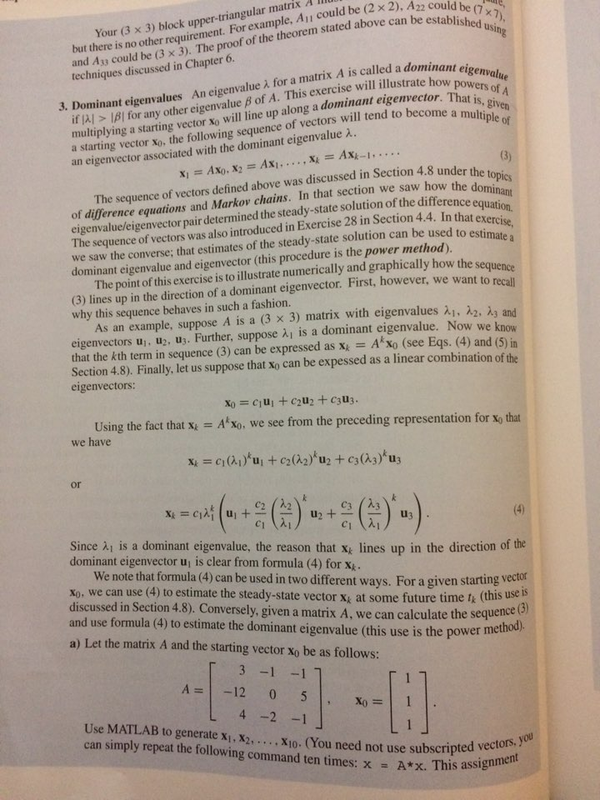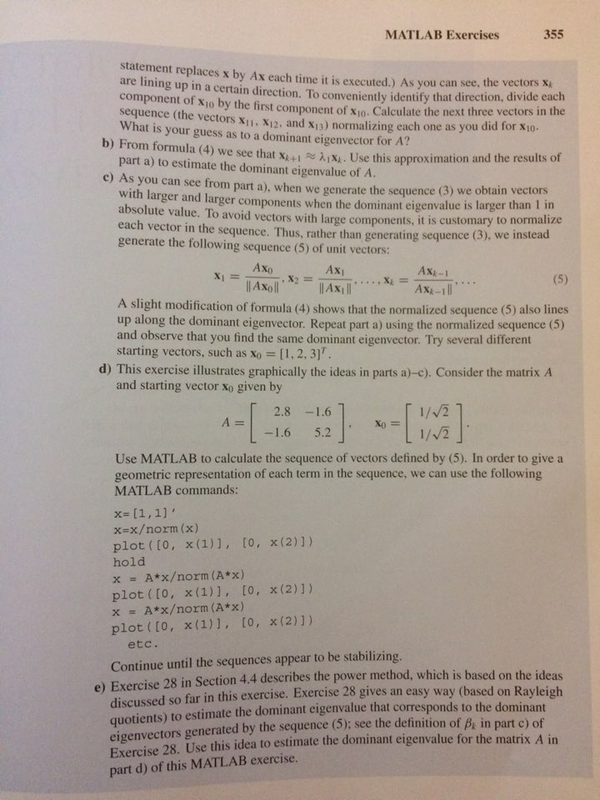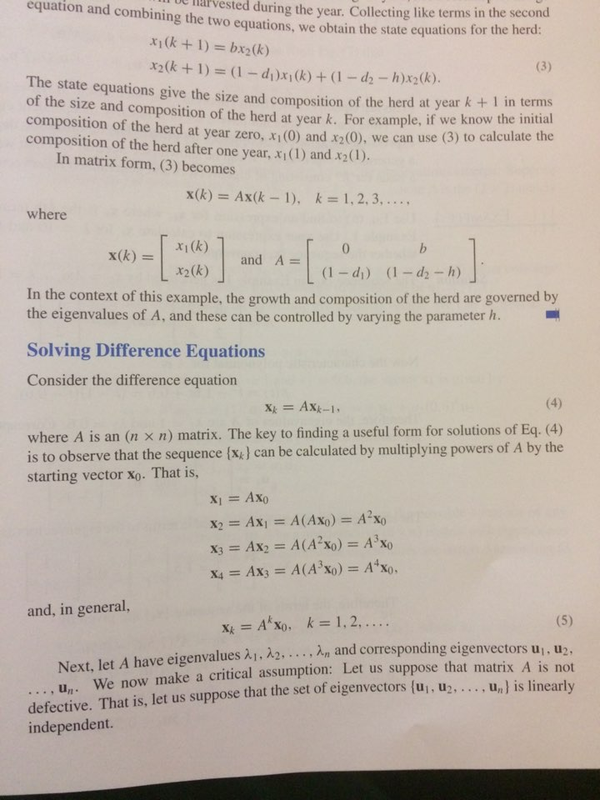# We Helped With This Algebra Homework: Have A Similar One?

SOLVEDCategory Math Algebra Undergraduate Solved Algebra Homework Help

## Short Assignment Requirements

I need to prepare 1 .m file and 1 PDF file for the answers.

## Assignment ImageYour (3 x 3) block upper-triangular matrix but there is no other requirement. For example, A11 could be (2 x 2). A22 could be (7 ×7), and A33 could be (3 x 3). The proof of the theorem stated above can be established u using techniques discussed in Chapter 6. 3. Dominant eigenvalues An eigenvalue for a matrix A is called a dominant eigenvalue if > BI for any other eigenvalue ß of A. This exercise will illustrate how powers of A multiplying a starting vector Xo will line up along a dominant eigenvector. That is, given a starting vector xo, the following sequence of vectors will tend to become a multiple of an eigenvector associated with the dominant eigenvalue A. (3) The sequence of vectors defined above was discussed in Section 4.8 under the topics of difference equations and Markov chains. In that section we saw how the dominant eigenvalue/eigenvector pair determined the steady-state solution of the difference equation. The sequence of vectors was also introduced in Exercise 28 in Section 4.4. In that exercise, we saw the converse; that estimates of the steady-state solution can be used to estimate a dominant eigenvalue and eigenvector (this procedure is the power method). The point of this exercise is to illustrate numerically and graphically how the sequence (3) lines up in the direction of a dominant eigenvector. First, however, we want to recall why this sequence behaves in such a fashion. = AX-1..... X₁ = Axo, X₂ = AX₁. ..., X = As an example, suppose A is a (3 x 3) matrix with eigenvalues A₁, A2, A3 and eigenvectors u₁, U₂, U3. Further, suppose à, is a dominant eigenvalue. Now we know Ak xo (see Eqs. (4) and (5) in that the kth term in sequence (3) can be expressed as X Section 4.8). Finally, let us suppose that xo can be expessed as a linear combination of the eigenvectors: or Xo = C₁U₁ + C₂U2 + C3U3. Using the fact that x = Axo. we see from the preceding representation for Xo that we have x = C₁ (A₁)*u₁ + c₂(22) u₂+ c3(23)*u3 x = (-+ () + (-) ). C3 X U₂ C1 A = = -12 Since A₁ is a dominant eigenvalue, the reason that x lines up in the direction of the dominant eigenvector u, is clear from formula (4) for X. We note that formula (4) can be used in two different ways. For a given starting vector Xo. we can use (4) to estimate the steady-state vector x at some future time 1 (this use is discussed in Section 4.8). Conversely, given a matrix A, we can calculate the sequence (3) and use formula (4) to estimate the dominant eigenvalue (this use is the power a) Let the matrix A and the starting vector xo be as follows: method). -1 3-1 0 5 H 4 -2 -1 Use MATLAB to generate X₁, X2..... X10. (You need not use can simply repeat the following command ten times: x= A*x. This assignment subscripted vectors, you (4) Xo =

## Assignment Imagestatement replaces x by Ax each time it is executed.) As you can see, the vectors X are lining up in a certain direction. To conveniently identify that direction, divide each component of X10 by the first component of X10. Calculate the next three vectors in the sequence (the vectors X11, X12, and X13) normalizing each one as you did for X10- What is your guess as to a dominant eigenvector for A? b) From formula (4) we see that X+1₁x. Use this approximation and the results of part a) to estimate the dominant eigenvalue of A. c) As you can see from part a), when we generate the sequence (3) we obtain vectors with larger and larger components when the dominant eigenvalue is larger than 1 in absolute value. To avoid vectors with large components, it is customary to normalize each vector in the sequence. Thus, rather than generating sequence (3), we instead generate the following sequence (5) of unit vectors: X₁ = Axo ||Axoll Ax₁ (5) ||AX||| A slight modification of formula (4) shows that the normalized sequence (5) also lines up along the dominant eigenvector. Repeat part a) using the normalized sequence (5) and observe that you find the same dominant eigenvector. Try several different starting vectors, such as Xo = [1, 2, 3]". A = -[ . X₂=== MATLAB Exercises 2.8 -1.6 -1.6 5.2 d) This exercise illustrates graphically the ideas in parts a)-c). Consider the matrix A and starting vector xo given by x= [1,1]' x=x/norm (x) plot ( [0, x(1)], [0, x(2)]) hold ***** X₁ = x = A*x/norm (A*x) plot ( [0, x(1)], [0, x(2)]) x = A*x/norm (A*x) plot ( [0, x(1)], [0, x(2)]) etc. 355 AX:-1 Ax-1|| Xo **** 1/√/2 1/√2 Use MATLAB to calculate the sequence of vectors defined by (5). In order to give a geometric representation of each term in the sequence, we can use the following MATLAB commands: Continue until the sequences appear to be stabilizing. e) Exercise 28 in Section 4.4 describes the power method, which is based on the ideas discussed so far in this exercise. Exercise 28 gives an easy way (based on Rayleigh quotients) to estimate the dominant eigenvalue that corresponds to the dominant eigenvectors generated by the sequence (5); see the definition of B, in part c) of Exercise 28. Use this idea to estimate the dominant eigenvalue for the matrix A in part d) of this MATLAB exercise.

## Assignment Imageequation and combining the two equations, we obtain the state equations for the herd: ested during the year. Collecting like terms in the second (3) x₁ (k+ 1) = bx₂(k) x2 (k+1)= (1-d₁)x₁(k) + (1 - d₂ - h)x2(k). The state equations give the size and composition of the herd at year k +1 in terms of the size and composition of the herd at year k. For example, if we know the initial composition of the herd at year zero, x₁ (0) and x2(0), we can use (3) to calculate the composition of the herd after one year, x₁ (1) and x₂ (1). In matrix form, (3) becomes where x(k)= [ x(k) = Ax(k-1), k = 1, 2, 3, .... X₁ (k) X2 (k) In the context of this example, the growth and composition of the herd are governed by the eigenvalues of A, and these can be controlled by varying the parameter h. \$] and, in general, and A = Solving Difference Equations Consider the difference equation 0 [a-a b)]. b (1-d₁) (1-d₂ - h) X} = AXk−1, where A is an (nx n) matrix. The key to finding a useful form for solutions of Eq. (4) is to observe that the sequence {x} can be calculated by multiplying powers of A by the starting vector xo. That is, X₁ = Axo X₂ = AX₁ = A (Axo) = A²xo X3 = AX2 = A(A2²xo) = A³xo X4 = AX3 = A (A³x0) = A¹x0, X = A xo, k = 1,2,.... (5) Next, let A have eigenvalues A₁, A2,..., An and corresponding eigenvectors u₁, U₂, ... un. We now make a critical assumption: Let us suppose that matrix A is not defective. That is, let us suppose that the set of eigenvectors (u₁, u₂..... u,) is linearly independent.

Is it free to get my assignment evaluated?

Yes. No hidden fees. You pay for the solution only, and all the explanations about how to run it are included in the price. It takes up to 24 hours to get a quote from an expert. In some cases, we can help you faster if an expert is available, but you should always order in advance to avoid the risks. You can place a new order here.

How much does it cost?

The cost depends on many factors: how far away the deadline is, how hard/big the task is, if it is code only or a report, etc. We try to give rough estimates here, but it is just for orientation (in USD):

 Regular homework \$20 - \$150 Advanced homework \$100 - \$300 Group project or a report \$200 - \$500 Mid-term or final project \$200 - \$800 Live exam help \$100 - \$300 Full thesis \$1000 - \$3000

How do I pay?

Credit card or PayPal. You don't need to create/have a Payal account in order to pay by a credit card. Paypal offers you "buyer's protection" in case of any issues.

Why do I need to pay in advance?

We have no way to request money after we send you the solution. PayPal works as a middleman, which protects you in case of any disputes, so you should feel safe paying using PayPal.

Do you do essays?

No, unless it is a data analysis essay or report. This is because essays are very personal and it is easy to see when they are written by another person. This is not the case with math and programming.

Why there are no discounts?

It is because we don't want to lie - in such services no discount can be set in advance because we set the price knowing that there is a discount. For example, if we wanted to ask for \$100, we could tell that the price is \$200 and because you are special, we can do a 50% discount. It is the way all scam websites operate. We set honest prices instead, so there is no need for fake discounts.

Do you do live tutoring?

No, it is simply not how we operate. How often do you meet a great programmer who is also a great speaker? Rarely. It is why we encourage our experts to write down explanations instead of having a live call. It is often enough to get you started - analyzing and running the solutions is a big part of learning.

What happens if I am not satisfied with the solution?

Another expert will review the task, and if your claim is reasonable - we refund the payment and often block the freelancer from our platform. Because we are so harsh with our experts - the ones working with us are very trustworthy to deliver high-quality assignment solutions on time.

## Popular Solved Assignments Like This

Customer Feedback

"Thanks for explanations after the assignment was already completed... Emily is such a nice tutor! "

Order #13073